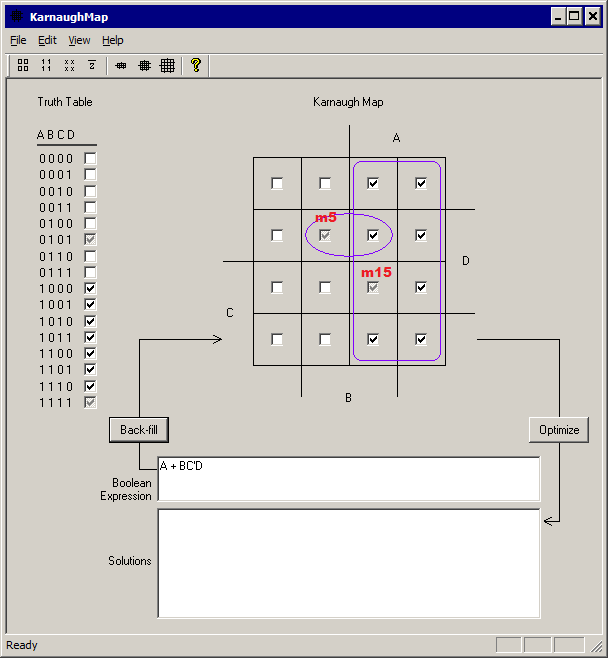## Handling the Don't Care conditionsKarnaughMap 4.4.5 (kmap445.exe) has the ability to automatically assign the best values to the don't care bits. In the example above, minterm bits m5 and m15 are don't cares. The values we assign to the don't care bits determine the complexity of the equation.

1. If we blindly assign 1 to both bits, the equation becomes B/CD + A, and this is not optimal.
2. If we blindly assign 0 to both bits, the equation becomes A/D + A/C + A/B, and this is not optimal.
3. If we assign 0 to m5 and 1 to m15, the equation becomes A, and this is optimal. Therefore this is the solution that KarnaughMap 3.2 will display at the top of the tree when you click on the Optimize button.

Kmap Home

Last update 08/02/2011